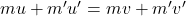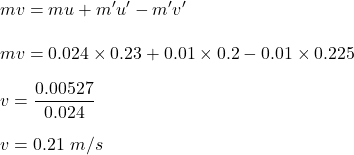## A 24.0 g marble moving to the right at 23.0 cm/s overtakes and collides elastically with a 10.0 g marble moving in the same direction at 20.

Question

A 24.0 g marble moving to the right at 23.0 cm/s overtakes and collides elastically with a 10.0 g marble moving in the same direction at 20.0 cm/s. After the collision, the 10.0 g marble moves to the right at 22.5 cm/s. Find the velocity of the 24.0 g marble after the collision. cm/s to the right?

in progress 0
6 months 2021-07-21T01:52:43+00:00 1 Answers 3 views 0

The final speed of the 24 g marble after the collision is 0.21 m/s.

Explanation:

Given that,

Mass of the marble 1, m = 24 g = 0.024 kg

Initial speed of the marble 1, u = 23 cm/s = 0.23 m/s

Mass of the marble 2, m’ = 10 g = 0.01 kg

Initial speed of the marble 2, u’ = 20 cm/s = 0.2 m/s

After the collision,

Final speed of marble 2, v’ = 22.5 cm/s = 0.225 m/s

We need to find the velocity of the 24.0 g marble after the collision. It is a case of elastic collision. Using the conservation of momentum as :v is the speed of  24.0 g marble after the collision.So, the final speed of the 24 g marble after the collision is 0.21 m/s.